# Nodal Analysis

## What is Nodal Analysis?

Nodal analysis is used for solving any electrical network, and it is defined as

The mathematical method for calculating the distribution of voltage between the nodes in a circuit.

This method is also known as the node-voltage method since the node voltages are with respect to ground. The following are the three laws that define the equation related to the voltage that is measured between each circuit node:

• Ohm’s law
• Kirchhoff’s voltage law
• Kirchhoff’s current law

### Features of Nodal Analysis

• Nodal analysis is an application of Kirchhoff’s current law.
• When there are ‘n’ nodes in a given electrical circuit, there will be ‘n-1’ simultaneous equations to be solved.
• To obtain all the node voltages, ‘n-1’ should be solved.
• The number of non-reference nodes and the number of nodal equations to be obtained are equal.

#### Recommended Video:## Procedure of Nodal Analysis

The following steps are to be followed while solving any electrical circuit using nodal analysis:

Step 1:

To identify the principal nodes and select one of them as a reference node. This reference node will be treated as the ground.

Step 2:

All the node voltages with respect to the ground from all the principal nodes should be labelled except the reference node.

Step 3:

The nodal equations at all the principal nodes except the reference node should have a nodal equation. The nodal equation is obtained from Kirchhoff’s current law and then from Ohm’s law.

Step 4:

To obtain the node voltages, the nodal equations can be determined by following Step 3.

Hence, for a given electrical circuit the current flowing through any element and the voltage across any element can be determined using the node voltages.

## Types of Nodes in Nodal Analysis

There are two types of nodes in nodal analysis:

• Non-reference node
• Reference node

### Non-Reference Node

The node that has a definite node voltage is known as a non-reference node.

### Reference Node

The node that acts as a reference point to all the other nodes is known as the reference node, which is also known as the datum node.

There are two types of reference nodes, and they are:

• Chassis ground

A type of reference node that acts as a common node for more than one circuit is known as chassis ground.• Earth ground

In any circuit when the earth potential is used as a reference, it is known as the earth ground.## What is Super Node?

The super node is defined as a voltage source that is connected between the two non-reference nodes such that these two nodes form a generalized node.

### Properties of Super Node

The following are the properties of super nodes:

• The difference between the voltage of two non-reference nodes can be determined at the super node.
• A super node does not have its own voltage.
• Kirchhoff’s current law and Kirchhoff’s voltage law, both are applied for solving the super node.

## Nodal Analysis Examples

Example 1:

For the following circuit, find Va by nodal analysis.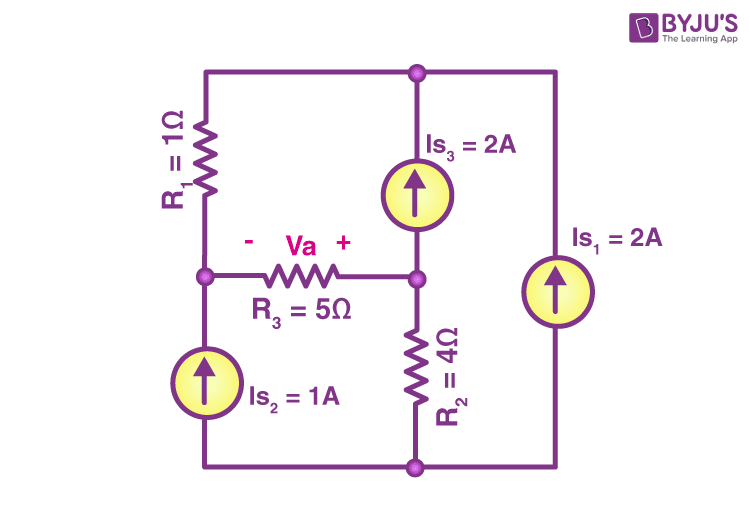Solution:

Step 1: To select a reference node and label the node voltages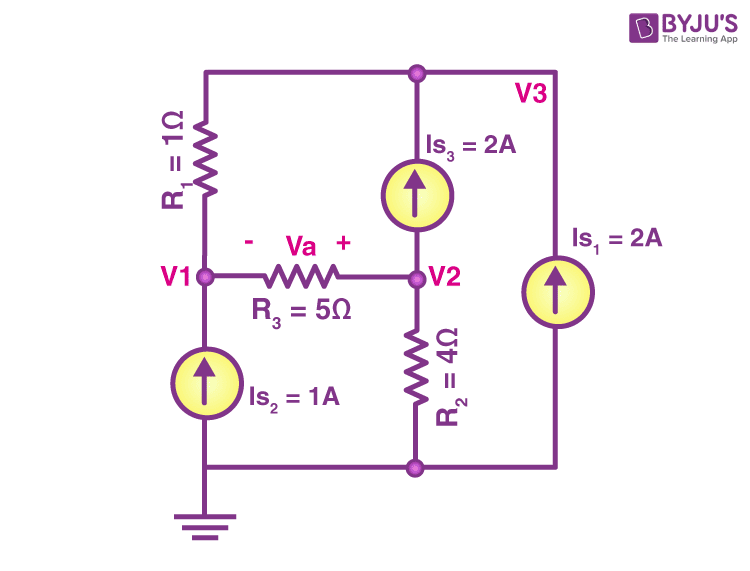Step 2: Apply Kirchhoff’s current law to all the nodes

Node 1:

$$-I_{s_{2}}+\frac{V_{1}-V_{2}}{R_{3}}+\frac{V_{1}-V_{3}}{R_{1}}=0\Rightarrow 6V_{1}-V_{2}-5V_{3}=5$$ (eq.1)

Node 2:

$$I_{s_{3}}+\frac{V_{2}-V_{1}}{R_{3}}+\frac{V_{2}}{R_{2}}=0\Rightarrow -4V_{1}+9V_{2}=-40$$ (eq.2)

Node 3:

$$-I_{s_{1}}-I_{s_{3}}+\frac{V_{3}-V_{1}}{R_{1}}=0\Rightarrow V_{3}-V_{1}=4$$ (eq.3)

From eq.1,2,3 we know that

V1 = 37v

V2 = 12v

V3 = 41

Step 3: To find the required quantities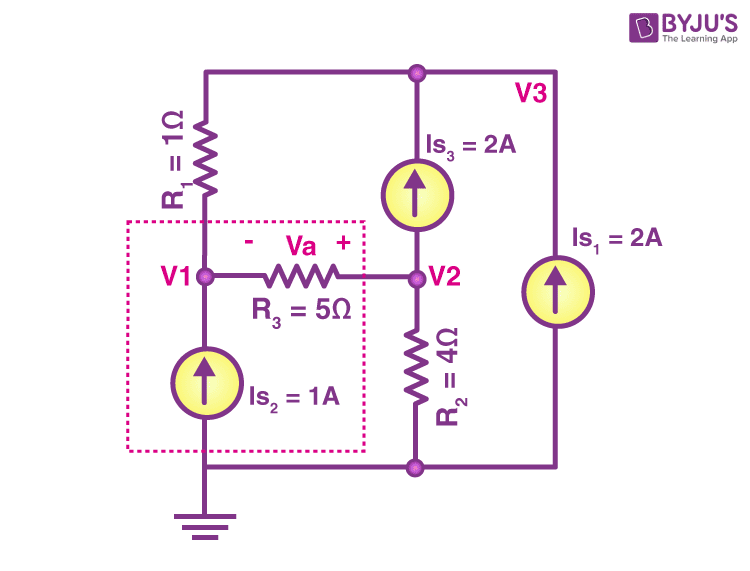Applying Kirchhoff’s voltage law in the above loop, we get

-V1 – Va + V2 = 0

Va = -25v

Example 2:

Determine the voltage at each node of the given circuit using nodal analysis.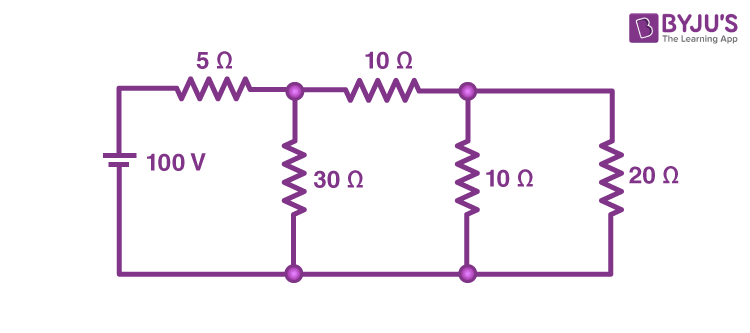Solution:

The number of nodes that are present in the given circuit is 3

The nodes that are present in the circuit are numbered as shown in the figure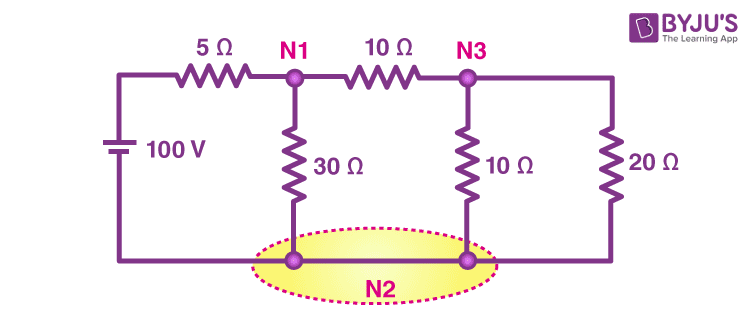Let node 2 be the reference node and the voltage of this node will be zero.

Using Kirchhoff’s current law at each node, we get

$$\frac{V_{1}}{30}+\frac{V_{1}-100}{5}+\frac{V_{1}-V_{3}}{10}=0$$ (eq.1)

This is a result of KCL at node 1

$$\frac{V_{3}-V_{1}}{10}+\frac{V_{3}}{10}+\frac{V_{3}}{20}=0$$ (eq.2)

This is a result of KCL at node 3

$$(\frac{1}{30}+\frac{1}{5}+\frac{1}{10})V_{1}-(\frac{1}{10})V_{3}=\frac{100}{5}$$ $$-(\frac{1}{10})V_{1}+(\frac{1}{10}+\frac{1}{10}+\frac{1}{20})V_{3}=0$$

Solving the above equations we get

V1 = 68.2v

V3 = 27.3v

## Frequently Asked Questions – FAQs

### If there are 12 nodes in a circuit, how many equations do we get?

We know that one node is always considered as a reference node, so the number of equations that we can get will always be less than the given number of nodes. Therefore, here the number of equations that can be obtained are 11.

### Which law is used in nodal analysis?

Kirchhoff’s current law is used in nodal analysis.

### What does Kirchhoff’s voltage law state?

According to Kirchhoff’s voltage law, the algebraic sum of the potential difference in any loop should be equal to zero.

### What does Kirchhoff’s current law state?

According to Kirchhoff’s current law, the current flowing into a node should be equal to the current flowing out of it.

### What is the advantage of nodal analysis?

The advantages of nodal analysis is that the number of equations required for determining the unknown quantities are minimum.